## Regular polychoron compounds

"I'm a Platonist - a follower of Plato - who believes that one didn't invent these sorts of things, that one discovers them. In a sense, all these mathematical facts are right there waiting to be discovered."
H. S. M. Coxeter.

There are, in total, 36 regular and 16 partially regular polychoron compounds. The fully regular compounds must have regular "cells" and vertex polyhedra; the difference is that either can be a regular polyhedron compound as well, in the discussion below we'll call attention to such cases. Like the regular star polychora, they must be stellations and facetings of the regular convex polychora because they must also be symmetric around each vertex and around each cell.

The partially regular compounds are stellations or facetings of a regular convex polychoron, but not both. In Figs. 16a and b, we have already seen two examples of the latter class:
1. The Compound of two Tesseracts can be represented by the model of the Tesseract in Fig. 16a. It is cell-regular, i.e., its 16 Cubic cells are in the same 3-D hyperplanes as those of the 16-cell, making it a stellation of that polychoron. It is not vertex regular: it has 24 vertices, 8 of each are common to both Tesseracts, eight touch only one of the Tesseracts, and another eight touch only the second Tesseract; it is therefore not a faceting of any regular convex polychoron.
2. Its dual, the Compound of two 16-cells, can be represented by the model of the 16-cell in Fig. 16b. By the rules of duality, this compound is vertex-regular, i.e. its 16 vertices are those of the Tesseract, making it a faceting of that polychoron. It is not cell regular: only 8 of its 24 cell planes are common to both 16-cells; it is therefore not a stellation of a regular convex polychoron.
There are nine additional Zomable compounds, all of them are fully regular. Below we show all their Zomable projections.

### Triality

Before proceeding, we present a brief explanation of the concept of Triality. In the case of duality in 3 dimensions, one can, by the definition of the meaning of duality, scale and rotate two concentric dual polyhedra A and B in such a way that each edge from A meets a perpendicular edge of B (see examples in Figs. 3a and b). If A and B are regular, their edges meet at their midpoints, as the cases depicted in Figs. 3a and b. Those two edges form a plane that is perpendicular to a third line joining the midpoint to the centers of A and B. Rectifying A and B results in the same rectified polyhedron (Fig. 4).

In the case of Triality in 4 dimensions, the situation is very similar, except that the perpendicular edges of three concentric "trial" polychora, A, B and C meet at right angles. A set of 3 16-cells is the only regular case; because the 3 16-cells are regular, their edges meet at their midpoints. These edges are in a 3-D "plane" that is itself perpendicular to a fourth direction, the line joining their meeting point to the centers of A, B and C. Rectifying all three polychora results in the same rectified polychoron, the regular case being the 24-cell, whose vertices coincide with the previous edge midpoints.

The same applies to projections: if we pick three "trial" projections of the 16-cell and rectify them, we obtain an invariant projection of the 24-cell. In this site, we show a triad of such trialities. The first we met already in Figs. 16a and b: the vertex-first and two cell-first projections of the 16-cell have the same rectification. The other two are shown in Figs. 28a and b below.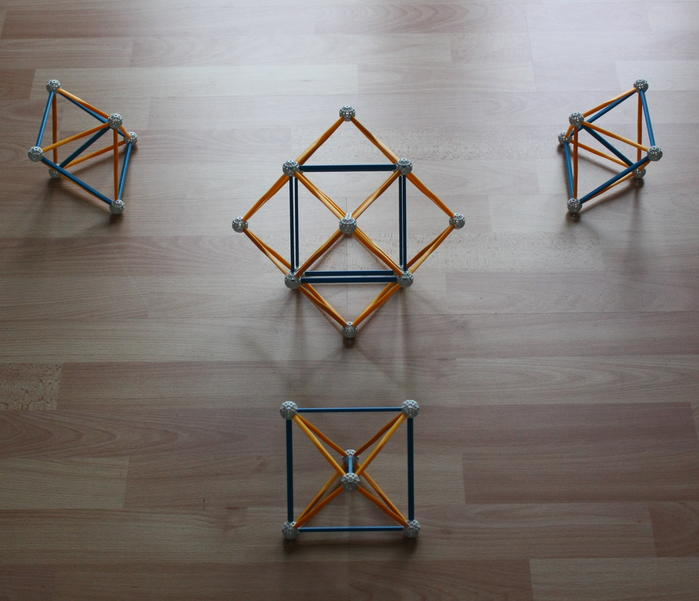Fig. 28a: Three edge-first projections of the 16-cell, with the central edges of the projections (very) loosely aligned along 3 perpendicular orthogonal axes in 3-d space. Rectifying these three projections, we obtain the vertex-first projection of the 24-cell in the middle. This is also known as its "pyritohedric" projection. It is the dual of the cell-first projection in Figs. 16a and b.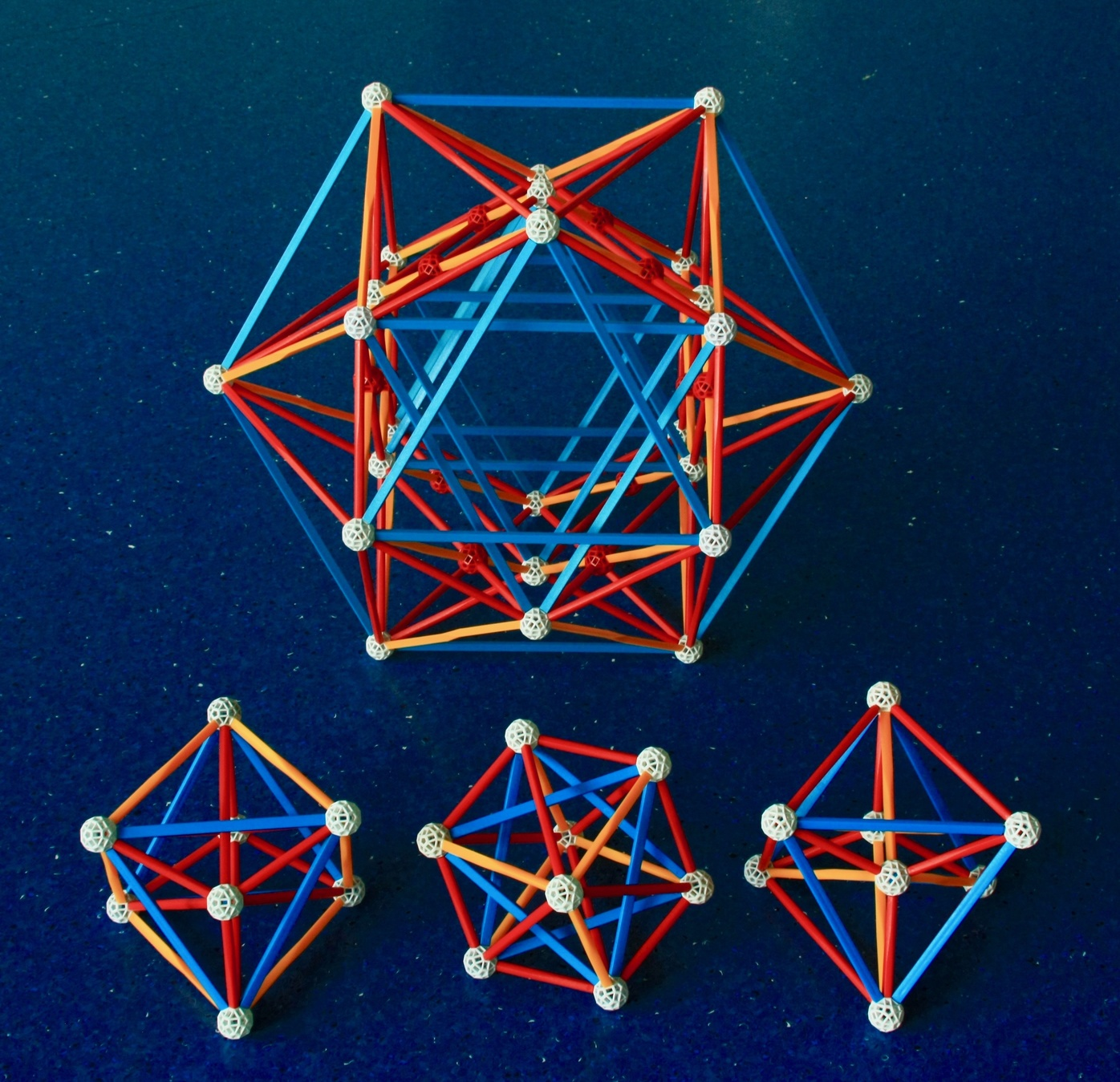Fig. 28b: Bottom: The three "prismatic" projections of the 16-cell. Rectifying all three projections results in the same projection of the 24-cell, also known as its "prismatic" projection, this is shown by the larger model above. The red balls represent edge intersections that happen only in the 3-d projection. One of the beauties of these models is that they show all vertices, edges, faces and cells separately, without superpositions.
For a detailed explanation of these models, see David Richter's Triality with Zometool page.

### Facetings of the 24-cell

The concept of triality allows a better understanding of the first object we discuss below, the Compound of three 16-cells. In the following Figures (29a, b and c), we show three projections of this compound, each of them a superposition of 3 "trial" projections of the 16-cell that appeared, respectively, in Figs. 16a and b, 28a and b. The edge midpoints, where they intersect by threes, are by analogy with Figs. 3a and b represented by the black balls. The real vertices of the compound are represented by the white balls.

Since the 24-cell is an invariant rectification of the three trial 16-cells, its vertices have the same arrangement as the edge midpoints of the Compound of three 16-cells. The same happens to its projections (see models on the left in each Figure), these have the same vertex arrangement (in white) as the edge midpoints of the associated projections of the Compound of three 16-cells (in black).

Importantly, there is a stellation operation, greatening, that transforms a 24-cell into a Compound of three 16-cells. As we've seen in Figs. 10a and b, this operation stellates Octahedra into a "Stella octangula". Greatening all the cells of the 24-cell, we obtain a Compound of three 16-cells. This has 3 × 16 = 48 Tetrahedral cells, which exist as "Stella octangula" pairs in the 3-D cell "planes" of the original 24-cell, with each Tetrahedron in the pair belonging to a different 16-cell.

Note that the greatening replaces each Triangular face of the original 24-cell by a larger dual Triangular face in the same plane (see Fig. 10a). In that Figure, we see that for each edge of the 24-cell there is a larger parallel edge of the new face that is twice as long. For this reason, for each strut in a Zomable projection of the 24-cell (see Figs, 29a, b and c on the left) there is a Zomable projection of the Compound of three 16-cells (on the right) with a parallel set of two struts of the same color and size in a straight line connected by a black ball.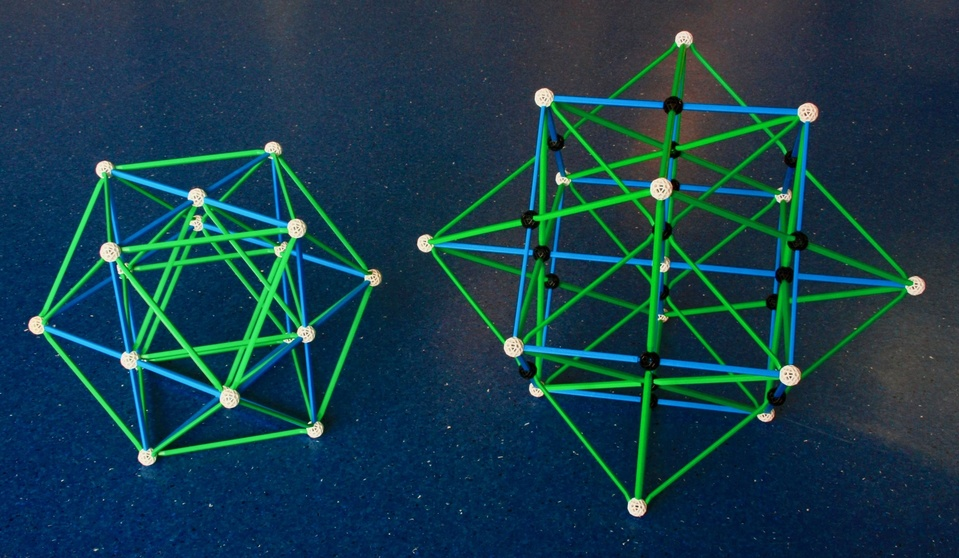Fig. 29a: Combining one vertex-first projection of the 16-cell in Fig. 16a with the projection of the Compound of two 16-cells in Fig. 16b, we obtain, on the right, the Octahedral projection of the Compound of three 16-cells. Since the projection of the 24-cell on the left has a Cuboctahedral envelope; its stellation's envelope is the first stellation of the Cuboctahedron: the composite of a Cube and a Octahedron in Fig. 3a.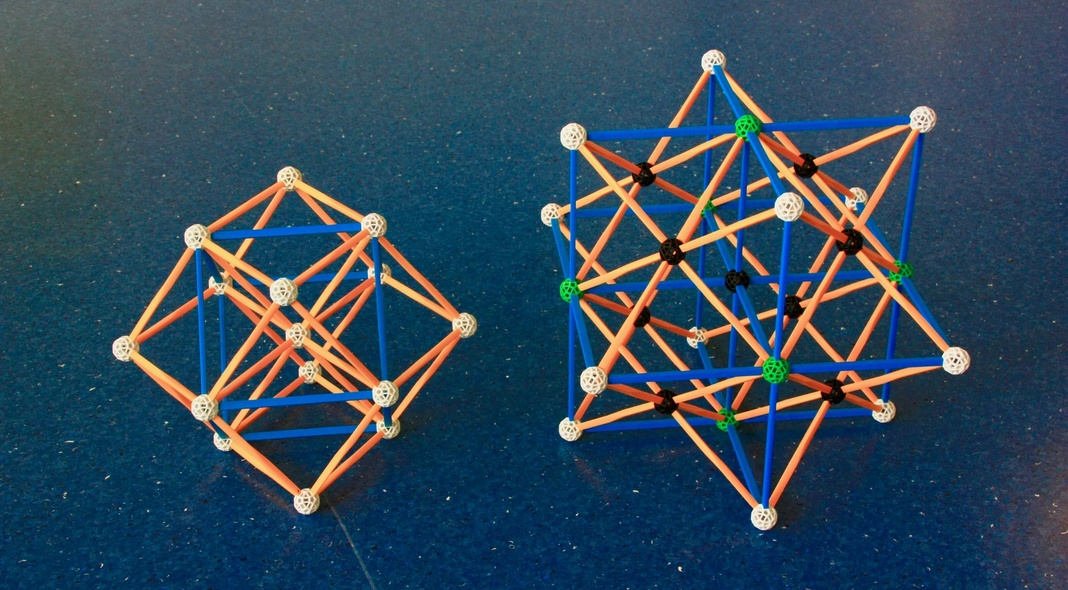Fig. 29b: Superposing the 3 orthogonal 16-cells in Fig. 28a, we obtain, on the right, a second Octahedral projection of the Compound of three 16-cells, its "pyritohedric" projection. Since the 24-cell on the left has the Rhombic dodecahedron as its outer envelope; its stellation's envelope is the first stellation of the Rhombic dodecahedron (see Figs. 13a and b); the latter is also known as "Escher's polyhedron".

This projection is centered on two superposed three-edge intersections, which are seen here without distortion. At the ends of those edges, we see real vertices (six pairs, superposed two by two) that are, in this model, depicted in green: the reason for this is that they coincide with 3-edge intersections; these are the intersections between the two blue edges we see crossing there with a third one (shortened by the projection to a point) that goes in between the two real vertices projected onto that same same green ball.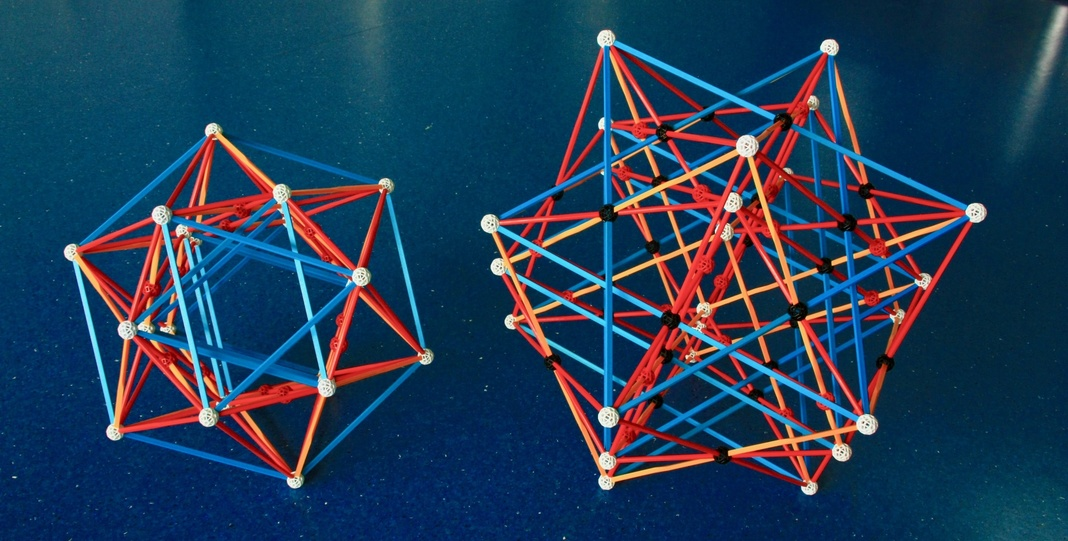Fig. 29c: Combining the three prismatic projections of the 16-cell in Fig. 28b one obtains, on the right, the prismatic projection of the Compound of three 16-cells. The red balls in both models are edge intersections that appear because of the projection; they are not real edge intersections in 4 dimensions.
Both models use size 3 struts, but this is not strictly necessary, as they can be built on a smaller scale. In the case of the Compound of three 16-cells, this would require 6 ultra-short red struts (R00), which are currently sold by Zometool.

How to build: Check David Richter's dedicated page, which also provides many more details on this model.

The real vertices of the projection of the Compound of three 16-cells in Fig. 29a have the same arrangement as the vertices of the projection of the 24-cell in Fig. 29b. Equally, the real vertices of its projection in Fig. 29b have the same arrangement as the vertices of the projection of the 24-cell in Fig. 29a, which is the dual to the projection of the 24-cell in Fig. 29b. This means that the Compound of three 16-cells is not only a stellation of a 24-cell, but also a faceting of its dual 24-cell.

It follows from this that the vertices of the prismatic projection of the Compound of three 16-cells in Fig. 29c are also the vertices of another projection of the 24-cell, the dual of the prismatic projection of the 24-cell in Fig. 29c. However, if you try to build it, you'll find that that projection is not Zomable. The same will logically apply to its greatening, a fourth projection of the Compound of three 16-cells, which has the same vertices as the prismatic projection of the 24-cell. This will have important consequences below.

This distinction between a 24-cell and its dual follows from an obvious, but important point: the vertices of a 24-cell and its dual are not in identical positions: in the models of the Compounds of three 16-cells, the former are shown in black, the latter in white, i.e., they are in two complementary sets of 24 points. The prismatic projection of the Compound of three 16-cells is especially good at showing all of these separately. The existence of two dual sets of 24-cell vertices implies that the same is true for the 3-D cell planes of two dual 24-cells.

***

It follows from this that the dual of the Compound of three 16-cells, the Compound of three tesseracts, is necessarily a faceting of a 24-cell and a stellation of its dual 24-cell, i.e., its cubic cells are not in the 3-D cell "planes" of the 24-cell being faceted. This should be kept in mind for what follows.

But what sort of faceting is it? As we saw above, any particular 24-cell (henceforth "A", for example, the 24-cell in Fig. 29a) can be greatened into a Compound of three 16-cells ("gA", Fig. 29a). Following the logic of Diagram IIb, the dual faceting transformation is equivalent to (if starting from A):
+ Finding dual (forming the dual 24-cell, "B", with faces centered on, and perpendicular to the edges of A, see Fig. 29b),
+ Greatening (forming gB, with faces in the same planes as those of B, see Fig. 29b),
+ Finding dual - forming fA, the Compound of three tesseracts.
The latter has 3 × 32 = 96 edges perpendicular to and going through the centres of the faces of gB and B. Therefore, they are at least partially coincident with the 96 edges of A. Since fA is a faceting of A, its vertex arrangement (the points where the edges end) is the same; therefore the edges of fA are exactly coincident with those of A (Fig. 29a).

As discussed after Diagram IIc, the dual operation to greatening is edge faceting, so it makes sense that the Compound of three tesseracts, the dual to the greatening of the dual 24-cell (see also Fig. 30) is an edge faceting of the 24-cell. Thus, the Compound of three tesseracts shares all Zometool representations of the 24-cell (shown in Figs. 29a, b and c). Two examples: the Octahedral projection of the 24-cell looks like three superposed face-first projections of Tesseracts (see detailed explanation in Fig. 30); the pyritohedric projection of the 24-cell looks exactly like a superposition of the Compound of two (vertex-first) Tesseracts (Fig. 16a) and the cell-first projection of the Tesseract (Fig. 16b).

Challenge to the reader: Locate the three Tesseracts in the prismatic projection of the 24-cell, and build Zometool models for them. How many Zometool models do you need? Are these the dual projections of the 16-cells in Fig. 28b or not?Fig. 30: Top right: The pyritohedric projection of the Compound of three 16-cells.
Bottom right: One of the components of the latter, the edge-first projection of the 16-cell.
Bottom left: Its dual, the face-first projection of the Tesseract. That central face is perpendicular to the central edge of the previous projection of the 15-cell.
Top left: The dual of the Compound of three 16-cells is another regular compound, with three Tesseracts. Amazingly, a projection of that regular compound (the dual of the pyritohedric projection of the Compound of three 16-cells) is well represented by this model of the cell-first projection of the 24-cell. With some attention, you might discern the 3 face-first Tesseracts in that model.

The Tesseracts in this compound have a total of 16 × 3 = 48 vertices, twice as much as the number of vertices of the compound. This implies that each vertex in the compound is shared by two Tesseracts. Thus, the vertex "polyhedron" of this compound consists of two vertex polyhedra of the Tesseract, which are Tetrahedra. Since the Compound of three tesseracts is an edge-faceting of the 24-cell, the two Tetrahedra of its vertex polyhedron form a faceting of the Cubic vertex polyhedron of the 24-cell, i.e., they are arranged as a Stella octangula (Fig. 10c). This has to be, since the dual Compound of three 16-cells has 24 Stellae octangulae as "cells".

There are no additional regular stellations and facetings of the 24-cell or the simpler regular convex polychora: all of them are compounds. This means that there are no additional regular star polychora.

The two regular facetings and stellations of the 24-cell are very important for understanding what follows. For each compound of n 24-cells below (with n = 5 and 25), we will see a projection of a compound of 3n Tesseracts, which is an edge faceting of the compound of n 24-cells and therefore represented by the same model (this will have a Stella octangula as vertex "polyhedron"), and a projection of a Compound of 3n 16-cells, which is a greatening (with twice the edge length, but the same edge directions) of the compound of n 24-cells; this will have n × 24 "Stellae octangulae" as composite "cells". Those models are made of multiples of the pyritohedric and prismatic projections of 24-cell or the Compound of three 16-cells above, with the prismatic projections being 4 times more numerous than the pyritohedric projections.

Because the duals of the prismatic projections are not Zomable, then none of the dual projections to the projections below are Zomable either, even if they represent a compound with another projection that is Zomable, or even of they represent exactly the same compound (as in the case of the self-dual Compound of five 24-cells - the projection dual to that of Figs. 31a and b is not Zomable). Please keep this in mind for the models below.

### Facetings of the 600-cell

Before we continue, a note: all remaining models suffer from the multiple blue intersections that we found with the models of the regular star polychora.

We have already seen some of the facetings of the 600-cell above: nine of the regular star polychora. Below we present projections of all remaining facetings of the 600-cell that are regular polychoron compounds. Like the 120-cell, 600-cell and regular star polychora, all these compounds have the full Hexacosichoric symmetry.

We start with the Compound of five 24-cells. The existence of this compound is a consequence of the fact, mentioned after Fig. 21, that the 120 vertices of the 600-cell can be decomposed in five sets of 24 vertices, each of them with the same vertex arrangement as a 24-cell.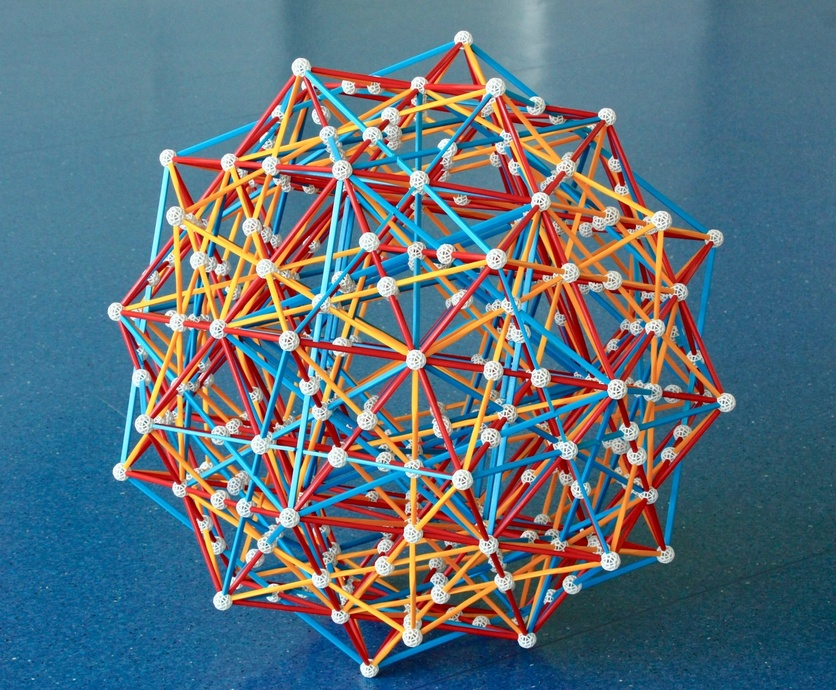Fig. 31a: This model represents a projection of the Compound of five 24-cells, here seen through a genuine three-fold rotational symmetry axis.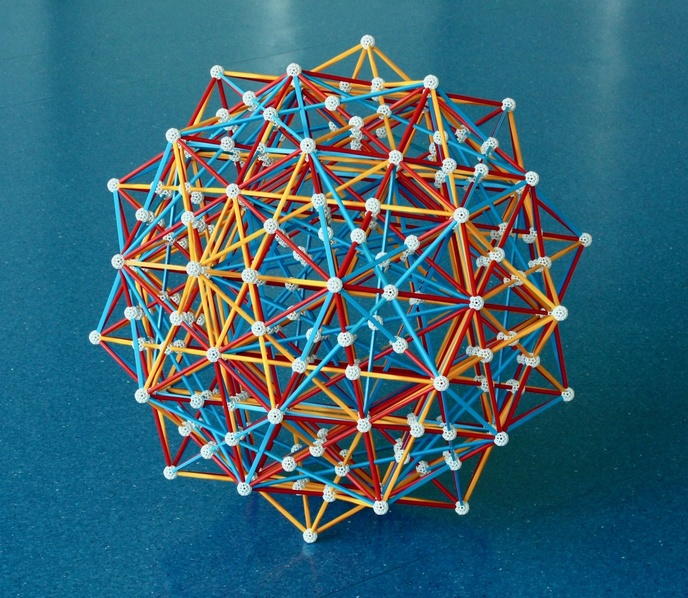Fig. 31b: The same projection as in the previous Figure, now seen through a special direction, the "Ghost symmetry" axis (see text).

See detailed explanation of this model in David Richter's dedicated page, which includes a part count. Here we're building this model on a φ smaller scale. This has an interesting implication: If we can find some size 3 struts (12 R3 and 12 Y3), we can use each of them to replace a combination of two struts and one ball where the ball is not strictly necessary (i.e., a ball where there is no intersection with other edges). Doing this, we can save 48 regular-sized struts and 24 balls compared to the part count in David Richter's page, and improve the appearance of the model.

This regular polychoron compound is one of the very few that are self-dual. Since this compound is a faceting of the 600-cell, its dual - in this case itself - is necessarily a stellation on the 120-cell.

In this projection, the vertex arrangement is the same as that of the Icosahedral projection of the 600-cell in Fig. 21. The whole projection has the same pyritohedral symmetry as the model of the irregular icosahedron and the Snub 24-cell in Figs. 18 and 19. This is unusual since the Compound of five 24-cells has full Hexacosichoric symmetry: for most other similarly symmetric objects, the only Zomable projections have Icosahedral symmetry. The reason for this is explained in the next page.

In this projection there are no axes of 5-fold symmetry, as we would naturally find in a model with Icosahedral symmetry. However, if we project this model into 2 dimensions along a special direction - as in Figure 31b - we see again a semblance of 5-fold symmetry. This is a remnant of the fact that the original compound can be inscribed in the 600-cell. The original paper describing this (Richter and Vorthmann, 2006) calls this phenomenon "Tenacious Symmetry". However, the name "Ghost symmetry" has gained wider acceptance, since it is more evocative. It is a widespread phenomenon, caused by the fact that the 2-D symmetries are rather "small": one can choose the vertices of a polychoron to project symmetrically into two dimensions, and have an intermediate 3-D projection that is still highly asymmetric. An extreme example are these two projections of the 600-cell.

***

This model also represents the Compound of fifteen tesseracts, which is obtained by edge-faceting the 24-cells of the previous compound. As discussed above, for the Compound of three tesseracts that results from each of those 5 24-cells, the 3-D planes of the Cubic cells are in the 3-D planes of the dual 24-cell. Therefore, the 15 × 8 = 120 Cubic cells of the compound of fifteen Tesseracts are in the same planes as the the cells of the dual of the Compound of five 24-cells, which is of course another Compound of five 24-cells. As we saw above, the latter is a stellation of the 120-cell, so the same applies to the Compound of fifteen tesseracts.

***

We now show the greatening of the Compound of five 24-cells and the dual of the Compound of fifteen tesseracts, the Compound of fifteen 16-cells.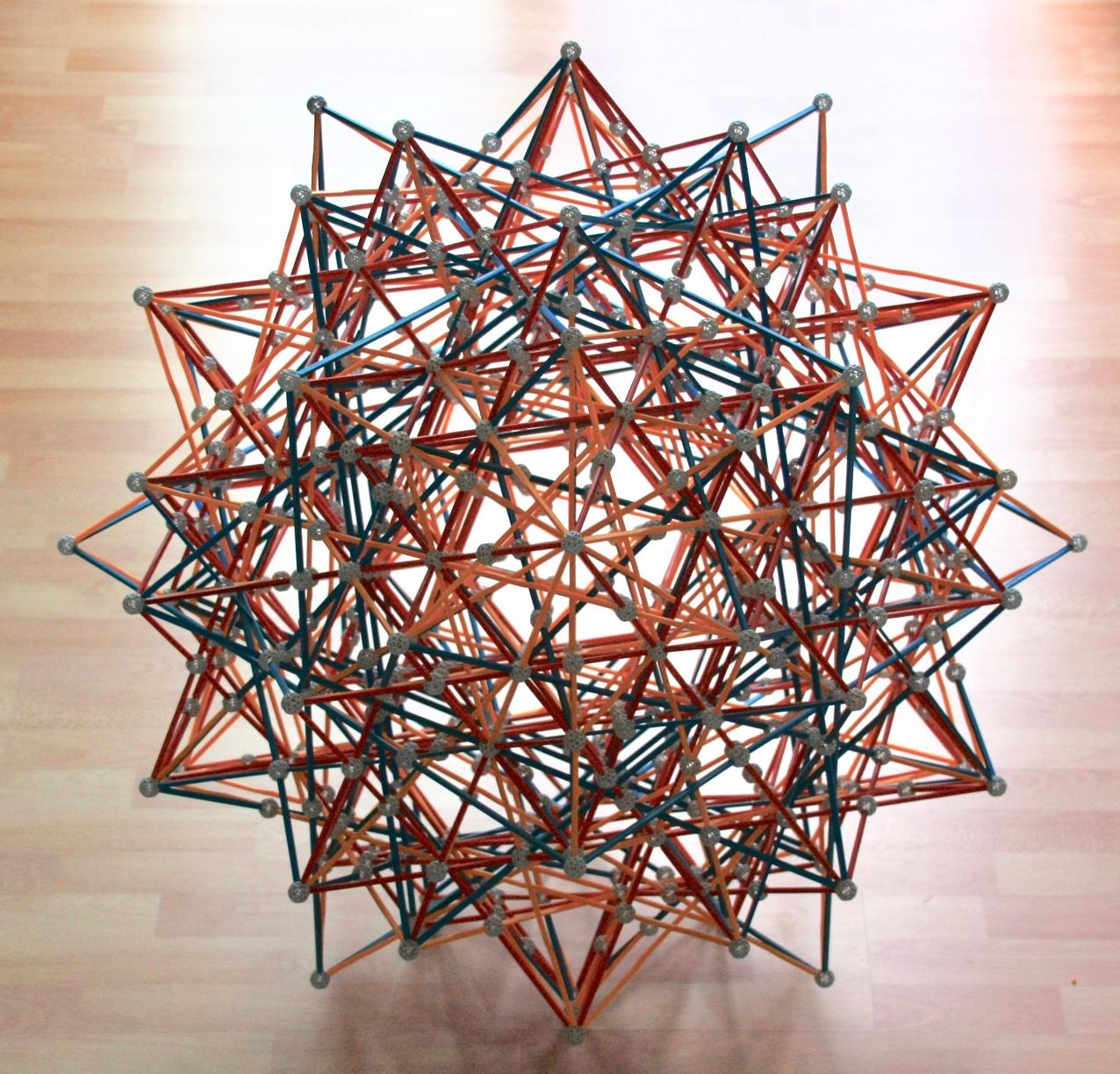Fig. 32a: A projection of the Compound of fifteen 16-cells. Here we see the model through a 3-fold rotatio/nal symmetry axis.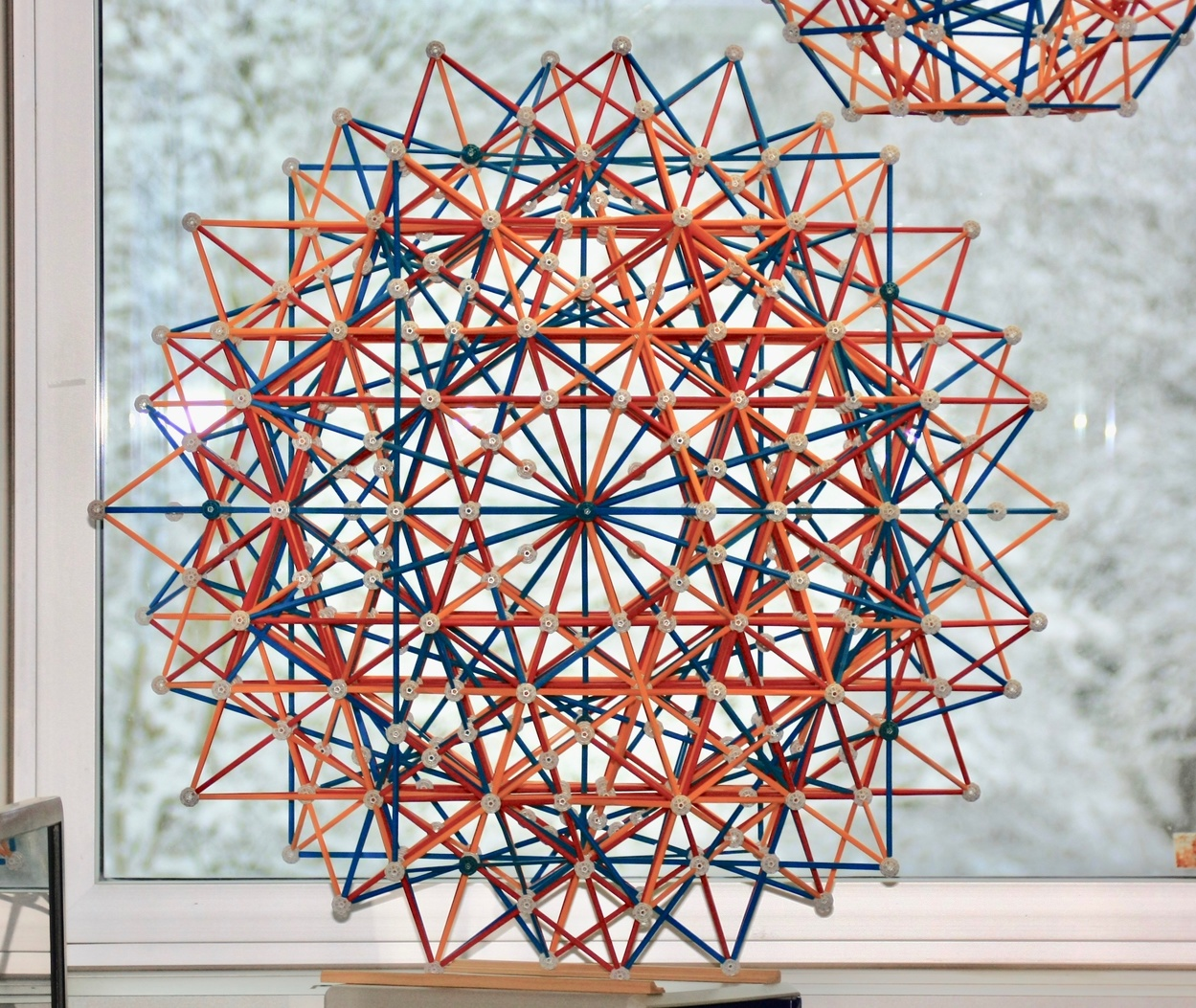Fig. 32b: The same model as in the previous Figure, here seen through a 5-fold "ghost symmetry" axis.

See also David Richter's dedicated page, which has a detailed description and part count. Because we're building the model on a φ smaller scale, we can use size 3 struts (in this case, 72 B3, 48 R3 and 120 Y3) to save 480 regular-sized struts and 240 balls from that part count, and greatly improve the appearance of the model. This cannot be built on a smaller scale: that would require yellow parts smaller than Y0, which have never been made by Zometool.

This particular projection is the greatening of the projection of the Compound of five 24-cells in Figs. 31a and b, therefore it has the same pyritohedric symmetry, despite the full Hexacosichoric symmetry of the Compound of fifteen 16-cells. The 24-cell in pyritohedric projection is greatened into a Compound of three 16-cells in pyritohedric projection (see Fig. 29b), and the four 24-cells in prismatic projection are greatened into four Compounds of three 16-cells in prismatic projection (see Fig. 29c).

Since greatenings preserve the 3-D cell "planes", then this compound is, like the Compound of five 24-cells, a stellation of the 120-cell. This has to be since its dual (the Compound of fifteen Tesseracts in Figs. 31a and b) is a faceting of the 600-cell. Since that compound is a stellation of the 120-cell, the Compound of fifteen 16-cells is a faceting of the 600-cell.

However, and uniquely in this projection, its vertex arrangement is not that of the Icosahedral projection of the 600-cell in Fig. 21, but instead the vertex arrangement of its Tetrahedral projection, shown in Fig. 37. This is a 1/5 subset of the vertex arrangement of the Icosahedral projection of the 120-cell in Fig. 22. This reflects a fundamental fact, that the 120-cell can be faceted by 600-cells, either five or ten (see Fig. 40). We will see a few important consequences of this below.

***

There are four regular compounds of twenty-five 24-cells. Among them, there is a dual pair with Hexacosichoric symmetry, where one of the compounds is a faceting of the 600-cell (see Fig. 33) and the other is a faceting of the 120-cell (Fig. 38). This implies that the former is a stellation of the 600-cell and the latter a stellation of the 120-cell.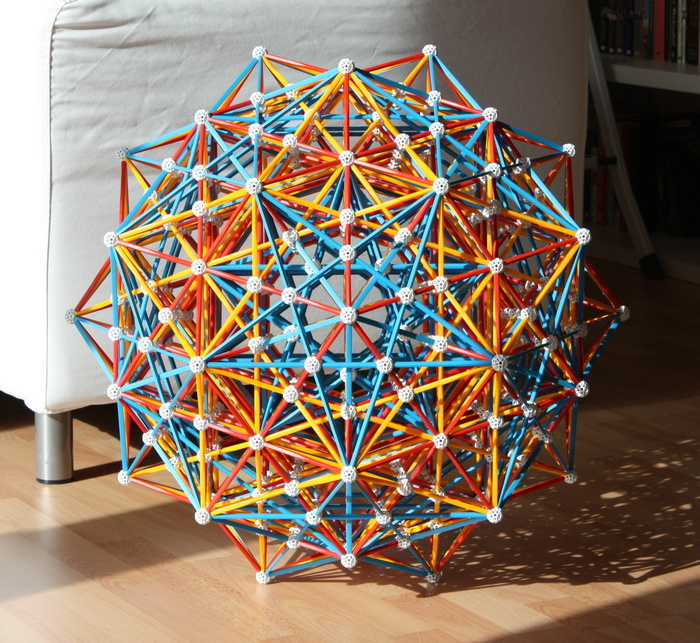Fig. 33: This model of the Icosahedral projection of the Stellated 120-cell and Great grand 120-cell, also represents the Icosahedral projections of two regular polychoron compounds: one of the Compounds of twenty-five 24-cells and one of the Compounds of seventy-five Tesseracts.

The model in this Figure can be built from the model of the Compound of five 24-cells in Figs. 31a and b by quintuplication (see Fig. 9b): To that projection, we add four more identical ones, each rotated around one of the "ghost symmetry" axes of the first compound by an additional 72 degrees. The resulting model has Icosahedral symmetry.

Since the resulting compound is still a faceting of the 600-cell, the quintuplication has not added any vertices to those of the Compound of five 24-cells: the 600 vertices of the 25 24-cells appear in the positions of the 120 vertices of the 600-cell. Thus each vertex is shared by five different 24-cells. Since the vertex polyhedron of the 24-cell is a Cube, the vertex "polyhedron" of this compound is a Compound of five cubes, which is a faceting of the Dodecahedral vertex polyhedron of the Stellated 120-cell. Thus the edge arrangement, which is defined by the overall vertex arrangement and the vertices of the vertex polyhedron, is that of the Stellated 120-cell. This means that this compound and the Stellated 120-cell are represented by the same Zometool model. Furthermore, since the Compound of five cubes shares the edge arrangement of the ditrigonal polyhedra, this compound shares the face arrangement of the aforementioned semi-regular ditrigonal polychora.

If you pay close attention to the way the quintuplication acts on the Compound of five 24-cells, you will notice something interesting: there is a duplication of edges. Indeed, the 25 × 96 = 2400 edges of the resulting Compound of twenty-five 24-cells cover the full set of 1200 edges of the Stellated 120-cell twice. This means that the edges of the 24-cells coincide in pairs. This is to be expected from the vertex "polyhedron": in the Compound of five cubes, each vertex is touched by two different Cubes.

***

This model also represents one of the regular Compounds of seventy-five Tesseracts, which is obtained by edge-faceting the 24-cells of the previous compound. We can also obtain this compound by quintuplication of the Compound of fifteen Tesseracts that is also represented by the model in Figs. 31a and b. The 16 × 75 = 1200 vertices of all these Tesseracts fall in sets of 10 on the 120 vertices of this compound. Since the vertex polyhedron of the Tesseract is a Tetrahedron, the vertex "polyhedron" of this compound is the Compound of ten tetrahedra*, which is also a faceting of the Dodecahedral vertex polyhedron of the Stellated 120-cell. Like the previous compound, the 75 × 32 = 2400 edges of the Tesseracts coincide in pairs. This is to be expected from the vertex "polyhedron": in the Compound of ten tetrahedra, each vertex is touched by two different Tetrahedra.

As discussed above, when we facet a 24-cell to obtain a Compound of 3 Tesseracts, the latter's cells are in the 3-D "planes" of a dual 24-cell. Thus, the 3-D cell "planes" of the Compound of seventy-five tesseracts in Fig. 33 are identical to those of the Compound of twenty-five 24-cells in Fig. 38, which is a stellation of the 120-cell. Thus, its 75 × 8 = 600 Cubic cells appear in sets of five in the cell planes of the 120-cell, i.e., this compound can be seen as a polychoron with 120 Compounds of five cubes as composite cells. You can see the central, undistorted and superposed projection of two of these Compounds of 5 cubes when you build this model. These Compounds of five cubes also highlight the undistorted vertex ``polyhedron" of the central vertex of the Compound of five 24-cells.

Because this Compound of seventy-five Tesseracts is an edge faceting of the Compound of twenty-five 24-cells in Fig. 33, its dual - a Compound of seventy-five 16-cells (see vZome virtual model in Fig. 39) - is a greatening of the dual Compound of twenty-five 24-cells in Fig. 38. That Compound of seventy-five 16-cells is necessarily a faceting of the 600-cell. It is the only such faceting that is not Zomable.

This ends the list of regular edge facetings of the Stellated 120-cell, and the list of Zomable projections of regular facetings of the 600-cell.

* When discussing the Compound of three tesseracts above, we said that other compounds of 3 n Tesseracts also have the Stella octangula as vertex figures. This is still true in this case, since the Compound of ten tetrahedra can be thought of as a Compound of five Stellae octangulae.

### Facetings of the 120-cell

There is a large number of regular and partially regular facetings of the 120-cell and their duals. These can be grouped in three classes depending on how they are derived:
1. The Great grand stellated 120-cell, the only regular polychoron in this list,
2. The largest group (39 regular and partially regular compounds) results from the fact, mentioned after Fig. 32b, that we can facet a 120-cell with 5 or 10 600-cells (see Fig. 40). This implies automatically that all facetings of the 600-cell (either 9 of the 10 regular star polychora or the regular polychoron compounds just mentioned above) can then be used to form new regular compounds that are also facetings of the 120-cell. The duals of all the partially regular facetings of the 120-cell derived in this process (like the Compounds of five and ten 600-cells themselves), which are partially regular stellations of the 600-cell, are also included in this group
3. The last group (3 regular compounds) arises from the fact that, unlike the 600-cell, the 120-cell can be faceted by 5-cells.
This list implies that the vertex arrangement of the 120-cell includes the vertex arrangements of all other regular polychora as subsets! This implies, by duality, that the 3-D cell planes of all regular polychora are subsets of the 3-D cell planes of the 600-cell.

In Figs. 26a and b, we showed a model of the single member of the first class. In Figs. 34a, b, c and 36a, b, we present the single regular compounds from the second and third classes that are Zomable (for an explanation on why this is the case, look here). It's nice that there are Zomable members of the three classes! However, as we see in those Figures, these models are extremely complex and require many parts. This is an unavoidable consequence of the large number of vertices of the 120-cell (600). Another thing is that these models cannot be made on a smaller scale, as that would require yellow struts shorter than Y0, which have never been made by Zometool.

The compounds represented by the Zometool models below have the full Hexacosichoric symmetry of the 600-cell and 120-cell; however, this is not true for all facetings of the 120-cell (like the Compound of five 600-cells, see Fig. 40).

***

We start with the single Zomable member of the second class, one of the three regular compounds of seventy-five 16-cells.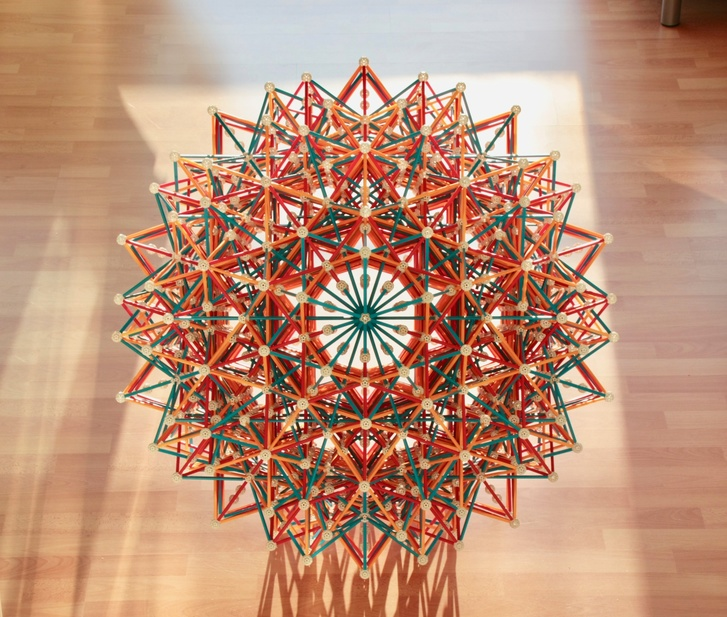Fig. 34a: The Icosahedral projection of the Compound of seventy-five 16-cells that facets the 120-cell and has full Hexacosichoric symmetry.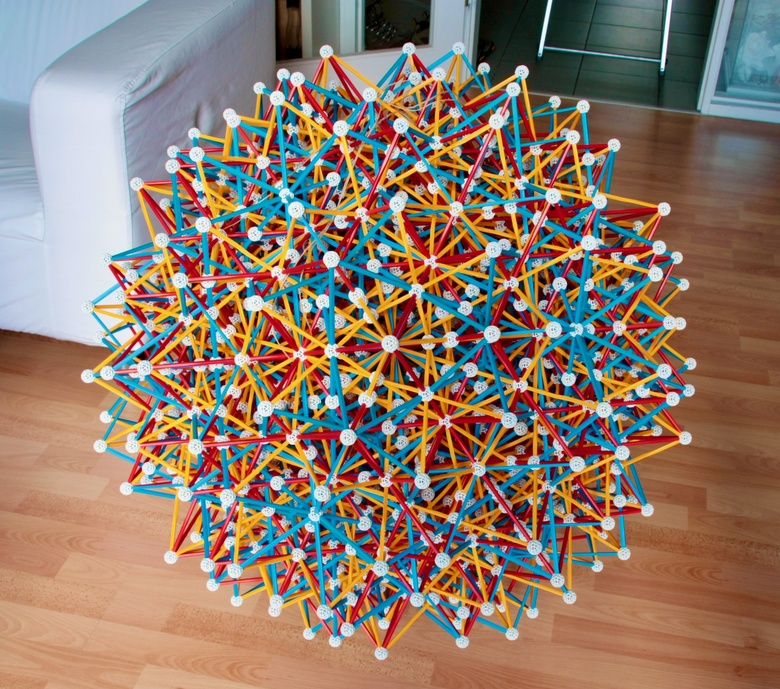Fig. 34b: The model is seen here from a 3-fold symmetry axis, and without counter-illumination.

To make this model, no size 3 struts are necessary!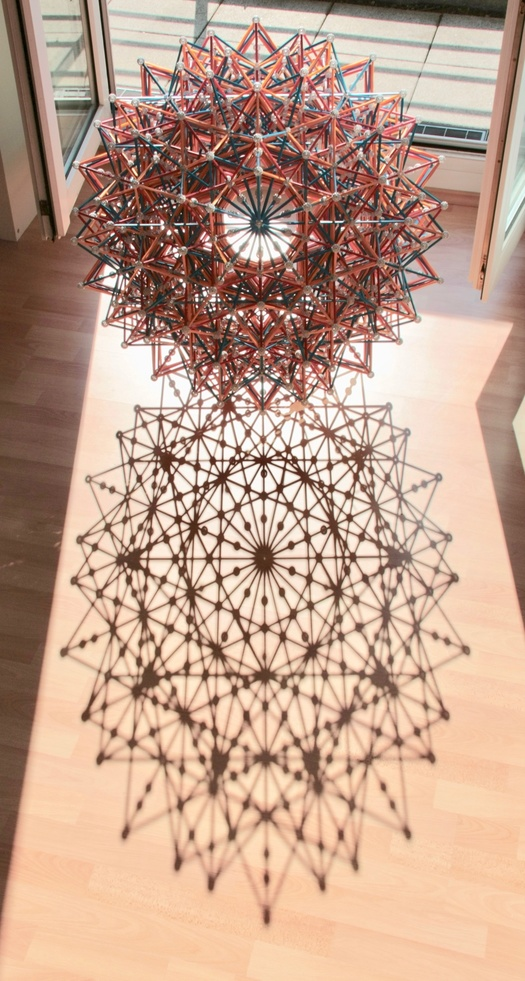Fig. 34c: When the Sun is at a good elevation, these models cast really nice shadows! Note the small bit of light right at the centre: that is the Sun shining through all the pentagonal holes of the nine balls in one of the six central axes of 5-fold symmetry of the model. This is proof that the parts in the model are aligned with very good precision. The model does not use size 3 struts, only commonly available sizes.

This compound is the greatening of the Compound of twenty-five 24-cells in Fig. 33. Since greatenings preserve the 3-D cell "planes", then this compound is also a stellation of the 600-cell. We can also derive this Compound from the Compound of fifteen 16-cells in Figs. 32a and b by quintuplication. Each of the five Compounds of fifteen 16-cells facets a 600-cell in Tetrahedral projection, five of which cover all the vertices of the 120-cell.

Because this Compound can be derived by greatening the Compound of twenty-five 24-cells in Fig. 33, its dual Compound of seventy-five tesseracts is an edge faceting of the dual Compound of twenty-five 24-cells in Fig. 38. Therefore both of those Compounds are represented by the same edge model.

***

Until now, all fully regular compounds we have built were Compounds of n 24-cells (with n = 1, 5, and 25) and compounds derived from them by edge-faceting (with 3n Tesseracts, which are represented by the same models) or greatening (with 3n 16-cells). That basically sums it up. However, the last model below represents something truly different.

Like the vast majority of regular compounds, none of the compounds represented above has the 5-cell as an element. In Fig. 15, we can see its vertex/cell first projection. In the Figure below, I show three more Zomable projections.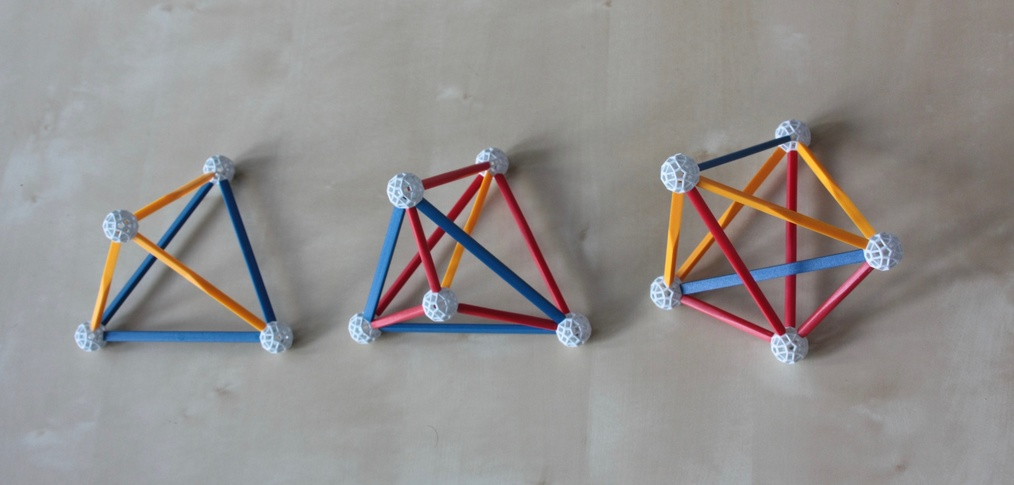Fig. 35: Three Zomable projections of the 5-cell.

In this page I name these from right to left, the "ghost-symmetric", "prismatic" and "pyritohedric" projections. The former was found by Scott Vorthmann, the other two were later found by David Richter. The name for the projection on the right comes from the fact that it has pentagonal ghost symmetry: from this perspective, we can see a Pentagram inscribed in a Pentagon. For a more complete description of this projection and its implications, see Scott's vZome page on this projection.

With these three projections, we can build a model of the Icosahedral projection of the Compound of 120 5-cells, this is shown below.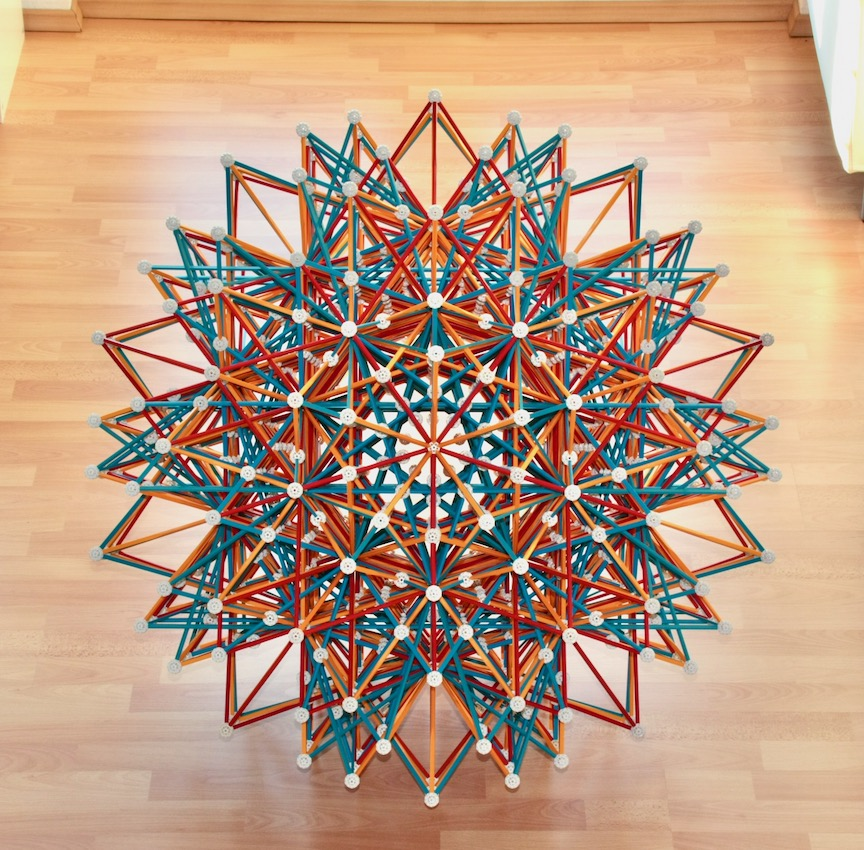Fig. 36a: The Icosahedral projection of the regular Compound of 120 5-cells with Hexacosichoric symmetry, here seen from a 5-fold symmetry axis.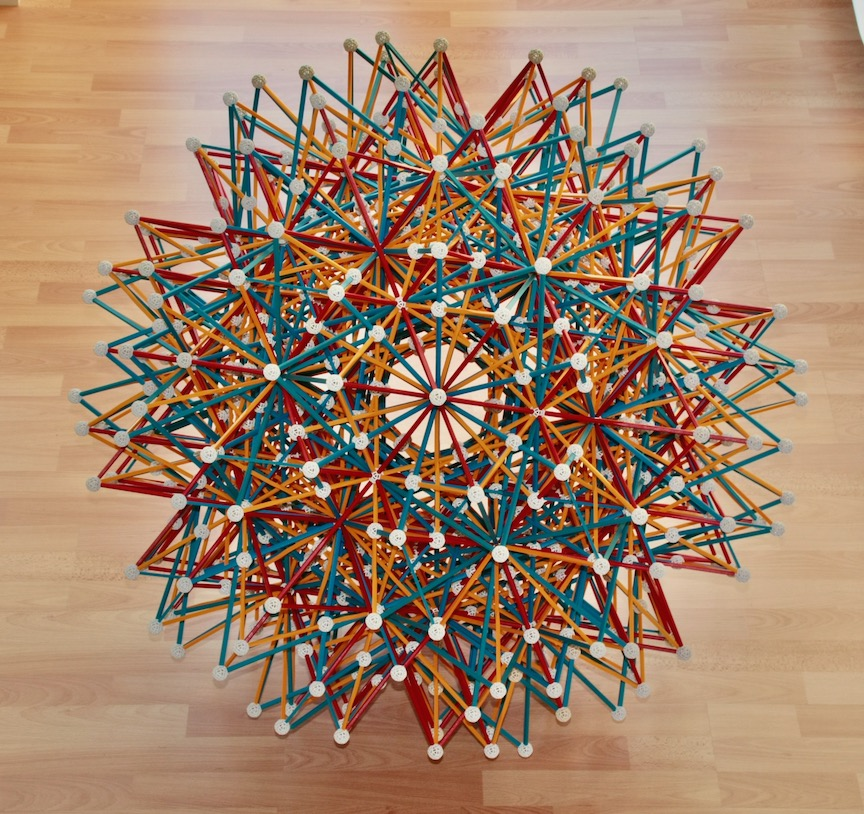Fig. 36b: Same model as in the previous Figure, seen here from a 3-fold symmetry axis.

To build this model at this scale (the outer vertices have the same separations as the models in Figs. 26a and b, and Figs. 34a, b and c), it is better to use 180 B3, 120 R3 and 260 Y3 struts. Doing this, you can save 560 balls and 1120 regular sized struts and greatly improve the appearance of the model.

This regular polychoron compound is special for several reasons. It is self-dual, a property it shares only with the Compound of five 24-cells (Figs. 31a and b) and the other two regular 5-cell compounds. Among the latter, only this compound is Zomable.

Since it is a faceting of the 120-cell, and it is made of 5-cells, it has 600 vertices with Tetrahedral vertex polyhedra, like the Great grand stellated 120-cell and the 120-cell. This and the fact that it is self-dual implies that it is a stellation of the 600-cell: like the Grand 600-cell, it has 600 Tetrahedral cells located in the same 3-D "planes" of the 600 Tetrahedral cells of the 600-cell. All of this makes it a 4-D analogue of the self-dual Compound of five tetrahedra.

The story behind this model is interesting. It is not in David Richter's list of Zometool projects, so I did not know whether the Compound of 120 5-cells was Zomable. However, when I saw the "ghost symmetric" projection of the 5-cell in Scott Vorthmann's vZome page on the 5-cell, I wondered whether it can be inscribed in the Icosahedral projection of the 120-cell (Fig. 22). I asked Scott about this, and he found out that the answer is "yes". Not only that, but the same is true for the other two projections of the 5-cell in Fig. 35.

Even more interestingly, he found that with only those three projections (20 pyritohedric, 40 prismatic and 60 ghost-symmetric) we can cover all the vertices of the projection of the 120-cell in Fig. 22. Thus, there is a Zomable projection of the Compound of 120 5-cells, with the same vertex arrangement as the model in Fig. 22 and therefore with the same Icosahedral symmetry! To demonstrate this, Scott made a very nice vZome model of the Icosahedral projection of this Compound, and Nan Ma (see star polytope page) made a Observable notebook on the Compounds of 120 5-cells. One of the cool things about this is that it also shows the dual projection.

David Richter then made us realize that the 120 × 10 = 1200 edges of the 120-cells in this compound can be obtained by extending the 1200 edges of the 120-cell and Stellated 120-cell - if we extend these further, we obtain the edges of the Great grand stellated 120-cell. This is important because it shows that the compound is isotoxal in 4-D (and therefore isohedral), i.e., this model really is a correct projection of the Compound of 120 5-cells. The parallelism to the edges of the 120-cell also necessarily their projections. Knowing this and having Scott's vZome model made the construction trivial. However, it should be noted that the Compound of 120 5-cells is not technically an edge stellation of the Stellated 120-cell; as we saw above, it is instead a stellation of the 600-cell.

When studying this compound, Nan Ma found a new regular, self-dual Compound of 720 5-cells. This was missed earlier in Coxeter's list of regular polychoron compounds! He then found out that Peter McMullen had discovered that regular compound in 2018! Not only that, but McMullen also discovered five other new regular compounds: a second Compound of 120 5-cells, a third Compound of seventy-five 16-cells, a third Compound of seventy-five Tesseracts, and a third and fourth partially regular Compounds of twenty-five 24-cells! All of these are facetings of the 120-cell.

That was a lot of fun!

As far as I know, this is the first physical model of this compound ever built. One has to build something very similar during the process of building the model of the Great grand stellated 120-cell in Figs. 26a and b, but there are some subtle differences that make it very unlikely anyone has built this exact model during that process.

This ends the list of the three Zomable regular facetings of the 120-cell, and our list of Zometool models of the regular compounds of regular polychora.

Main polytope page. Next: Zomability.